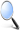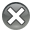Edelweiss Applied Science and Technology is an open access journal, our journal has a wide scope of topics related to science and technology, which are not limited to Basic Science, life science, computer science, environmental science, chemical science, electrical science, electronic engineering, industrial engineering, material science, astronomy and astrophysics.

# Edelweiss Applied Science and Technology (ISSN: 2576-8484)Research Article :

## The Helmholtzian Operator and Maxwell-Cassano Equations of an Electromagnetic-nuclear Field

Claude Michael Cassano

### Abstract

This article demonstrates that when acting on a four-vector doublet, the Helmholtzian may be factored into two 4×4/8×8 differential matrices, resulting in a four-vector doublet Klein-Gordon equation with source. This factorization enables yielding of a mass-generalized set of Maxwell s equations.

### Introduction

The Helmholtz differential equation [1-3] is a linear second order differential equation, generalization of the wave equation. A Helmholtzian operator is a linear second order differential operator, typically in four independant doublet-variables; a generalization of the dAlembertian operator  where its additional constant vanishes. When the time-independant version (in three independent (space) variables acting on a function or vector vanishes, the resulting equation is called the Helmholtzs equation. In four dimensions this equation is referred to as the Klein-Gordon equation [5-9] (with imaginary constant).

(Because the Klein-Gordon equation is the four-dimensional generalization of the three-dimensional Helmholtz equation; and each, of course may be generalized to higher dimensions, I have chosen to denote the operator with the Helmholtz designation).

This article demonstrates that when acting on a four-vector doublet, the Helmholtzian may be factored into two 4×4/8 × 8 differentials matrices in two distinct ways, as follows:

It doesnt take much more than a cursory look to see that this Helmholtzian operator and factorization is a generalization of the dAlembertian operator and its factorization .

The four-vector-doublet Klein-Gordon equation may be written as a matrix product. This, thus, when operated on a four-vector-doublet, gives a matrix product definition for the Helmholtzian operator. (The scalar Klein-Gordon equation is a special case of this four-vector-doublet version, where there are three restrictions on the four component-doublets of the potential A , leaving the single independent function-pair.)(Note that the Dirac equation [5-9] is a set of equations on a scalar doublet; so there is, in this way, Helmholtzian factorization consistency.)(And, there is a deeply intimate relationship with the Dirac equation).

Another great thing about using this description of the Helmholtzian is that if the matrix is applied to the column vector, the result may be expressed in terms of generalized E and B vector components associated with generalized electromagnetic field (with the appropriate definitions of A0 and x0 )(just as with the dAlembertian operator). And, when the final matrix is applied, the result is mass-generalized.

Maxwells inhomogeneous field equations [10-15] (with the gauge fixing term)(because the homogeneous field equations are identities, which actually appear as such in the final computation by all those terms canceling each other out). So, this is an incredibly compact way of writing both the Klein-Gordon equation and mass-generalized Maxwells equations of an electromagnetic-nuclear field [10-15].

Additionally, the above process may be generalized for any dimension power of two, thus generalizing the Helmholtzian (and, thus the dAlembertian) as well as the mass-generalized Maxwells equations to any dimension power of two (using the weighted matrix product to construct suitable algebras and doing the same analysis on it).

Comparing to the above  dAlembertian factorization, a mass-generalization of Maxwells equations is a clear result.

### References

1.        Abramowitz M and Stegun I. Handbook of Mathematical functions with Formulas, Graphs and Mathematical Tables (1964) Dover Publications, New York, USA.

2.        Sommerfeld A. Partial Differential Equations in Physics (1949) Academic Press, New York, USA.

4.        Cassano CM. The dAlembertian operator and Maxwells equations (2018) J Mod Appl Phys 2: 26-28.

5.        Ryder LH. Quantum Field Theory (2nd Ed) (2008) Cambridge University Press, Cambridge, UK.

6.        Merzbacher,Eugen; Quantum Mechanics, 3rd Ed (1997) John Wiley, USA

7.        Hannabuss K. An Introduction to Quantum Theory (1997) Clarendon Press, UK.

8.        Schiff LI and Bandhyopadhyay. Quantum Mechanics (2014) McGraw-Hill, Inc, USA

10.     Kovetz A. Electromagnetic Theory (2000) Oxford University Press, Inc., New York, USA.

11.     Woan G. The Cambridge Handbook of Physics Formulas (2000) Cambridge University Press, New York, USA.

12.     Feynman RP. The Feynman Lectures on Physics (2011) Addison–Wesley, USA 2.

13.     Hazewinkel M. "Maxwell equations", Encyclopedia of Mathematics (1994) Springer Science Business Media, Kluwer Academic Publishers, Netherlands.

14.     Cook DM. The Theory of the Electromagnetic Field (2002) Courier Dover Publications, New York, USA.Keywords